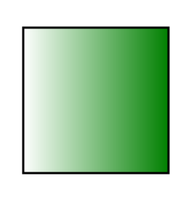Related Articles
HTML SVG Gradients
• Last Updated : 24 Aug, 2020

SVG Gradient is used to smooth transition one color to another color within a shape. SVG provides two types of gradients.

• Linear Gradients: Transition from one direction to another.
• Radial Gradients: Transition circularly from one color to another from one direction to another.

Linear Gradients: The linear-gradient() CSS function is used to create an image which consist a progressive transition between two or more colors along a straight line.

Syntax:

<linearGradient
gradientUnits =”units to define contents of gradient”
gradientTransform = “definition of an additional transformation
from the gradient coordinate system onto the target coordinate system”

x1=”x-axis co-ordinate”
y1=”y-axis co-ordinate”
x2=”x-axis co-ordinate”
y2=”y-axis co-ordinate”

spreadMethod=”indicates method of spreading the gradient within graphics element”
xlink:href=”reference to another gradient” >
</linearGradient>

Attribute:

• gradientUnits: Units to define the coordinate system for the various length values within the gradient.
• x1: x-axis co-ordinate of the gradient vector.
• y1: y-axis co-ordinate of the gradient vector.
• x2: x-axis co-ordinate of the gradient vector.
• y2: y-axis co-ordinate of the gradient vector.
• spreadMethod: Indicates method of spreading the gradient within graphics element. Default is ‘pad’.
• xlink:href: Used to refer to another gradient.

Example:

 ```<``html``>`` ` `<``body``>``    ``<``svg` `width``=``"400"` `height``=``"400"``>``        ``<``defs``>``            ``<``linearGradient` `id``=``"GFGGradient"``>``                ``<``stop` `offset``=``"0%"` `stop-color``=``"white"` `/>``                ``<``stop` `offset``=``"100%"` `stop-color``=``"green"` `/>``            ````        ```` ` `        ``<``g``>``            ``<``rect` `x``=``"100"` `y``=``"100"` `width``=``"200"` `                ``height``=``"200"` `stroke``=``"black"` `                ``stroke-width``=``"3"` `fill``=``"url(#GFGGradient)"` `/>``        ````    `````` ` ``

Output:Radial Gradients: The radial-gradient() CSS function is used to create an image which consist of a progressive transition between two or more colors that radiate from an origin. Its shape may be a circle or an ellipse.

Syntax:

<radialGradient
gradientUnits =”units to define co-ordinate system of contents of gradient”
gradientTransform = “definition of an additional transformation
from the gradient coordinate system onto the target coordinate system”

cx=”x-axis co-ordinate of center of circle.”
cy=”y-axis co-ordinate of center of circle.”

r=”radius of circle”

fx=”focal point for the radial gradient”
fy=”focal point for the radial gradient”

spreadMethod=”indicates method of spreading the
gradient within graphics element”
xlink:href=”reference to another gradient” >
</radialGradient>

Attribute:

• gradientUnits: Units to define the coordinate system for the various length values within the gradient.
• cx: x-axis co-ordinate of the center
• cy: y-axis co-ordinate of the center
• r: Radius of the center.
• fx: Focal point of radial gradient.
• fy: focal point of radial gradient.
• spreadMethod: Indicates method of spreading the gradient within graphics element. Default is ‘pad’.
• xlink:href: Used to refer to another gradient.

Example:

 ```<``html``>`` ` `<``body``>``    ``<``svg` `width``=``"400"` `height``=``"400"``>``        ``<``defs``>``            ``<``radialGradient` `id``=``"GFGGradient"``>``                ``<``stop` `offset``=``"0%"` `stop-color``=``"white"` `/>``                ``<``stop` `offset``=``"100%"` `stop-color``=``"green"` `/>``            ````        ```` ` `        ``<``g``>``            ``<``rect` `x``=``"100"` `y``=``"100"` `width``=``"200"` `                ``height``=``"200"` `stroke``=``"green"` `                ``stroke-width``=``"3"` `fill``=``"url(#GFGGradient)"` `/>``        ````    `````` ` ``

Output:My Personal Notes arrow_drop_up